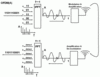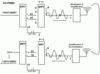# An Introduction To SC-FDMA Used By LTE In Uplink Direction

Both WiMAX and the UMTS successor technology LTE use Orthogonal Frequency Division Multiplexing (OFDM) as the core modulation technology on the air interface in downlink direction. In uplink direction, however, the two systems go different ways. While WiMAX uses OFDMA (Orthogonal Frequency Division Multiple Access), the 3GPP (3rd Generation Partnership Project) standardization group has decided to use SC-FDMA (Single Carrier Frequency Division Multiple Access) instead.

In essence, SC-FDMA builds on OFDMA so the two systems are not as different as it seems at first. In addition, the abbreviation SC-FDMA is quite misleading as the technology, like OFDMA, also uses many sub-carriers on the air interface. To explain how SC-FDMA works it’s best to first take a look at OFDMA (used by WiMAX) and then discuss the differences to SC-FDMA.

OFDMAOFDMA transmits a data stream by using several narrow band sub-carriers simultaneously, e.g. 512, 1024 or even more depending on the overall available bandwidth (e.g. 5, 10, 20 MHz) of the channel. As many bits are transported in parallel, the transmission speed on each sub carrier can be much lower than the overall resulting data rate. This is important in a practical radio environment in order to minimize effect of multipath fading created by slightly different arrival times of the signal from different directions.

As shown in the first figure on the left the input bits are first grouped and assigned for transmission over different frequencies (sub-carriers). In the example, 4 bits (representing a 16QAM modulation) are using for constructing each sub-carrier. In theory, each sub-carrier signal could be generated by a separate transmission chain hardware block. The output of these blocks would then have to be summed up and the resulting signal could then be sent over the air. Due to the high number of sub-carriers used this approach is not practicable. Instead, a mathematical approach is taken: As each sub-carrier is transmitted on a different frequency a graph which shows the frequency on the x-axis and the amplitude of each sub-carrier on the y-axis can be constructed. Then, a mathematical functional called Inverse Fast Fourier Transformation (IFFT) is applied which transforms the diagram from the frequency domain to time domain. This diagram has the time on the x-axis and represents the same signal as would have been generated by the separate transmission chains for each sub-carrier when summed up. The IFFT thus does exactly the same as the separate transmission chains for each sub carrier would do including summing up the individual results.

On the receiver side the signal is first demodulated and amplified. The result is then treated by a Fast Fourier Transformation function which converts the time signal back into the frequency domain. This reconstructs the frequency/amplitude diagram created at the transmitter. At the center frequency of each sub-carrier a detector function is then used to generate the bits which were originally used to create the sub-carrier.

SC-FDMADespite its name, Single Carrier Frequency Division Multiple Access (SC-FDMA) also transmits data over the air interface in many sub-carriers but adds an additional processing step as shown in the second figure. Instead of putting 4 bits together as in the OFDM example to form the signal for one sub-carrier, the additional processing block in SC-FDMA spreads the information of each bit over all the sub-carriers. This is done as follows: Again, a number of bits (e.g. 4 representing a 16 QAM modulation) are grouped together. In OFDM, these groups of bits would have been the input of the IDFT. In SC-FDMA, however, these bits are now piped into a Fast Fourier Transformation (FFT) function first. The output of the process is the basis for the creation of the sub-carriers for the following IFFT. As not all sub-carriers are used by the mobile station, many of them are set to zero in the diagram. These may or may not be used by other mobile stations.

On the receiver side the signal is demodulated, amplified and treated by the Fast Fourier Transformation function in the same way as in OFDMA. The resulting amplitude diagram, however, is now not analyzed straight away to get the original data stream but fed to the Inverse Fast Fourier Transformation function to remove the effect of the additional signal processing originally done at the transmitter side. The result of the IFFT is again a time domain signal. The time domain signal is now fed to a single detector block which recreates the original bits. Thus, instead of detecting the bits on many different sub-carriers, only a single detector is used on a single carrier.

Summary of the difference between OFDM and SC-FDMA:

OFDM takes groups of input bits (0’s and 1’s) to assemble the sub-carriers which are then processed by the IDFT to get a time signal. SC-FDMA in contrast first runs an FFT over the groups of input bits to spread them over all sub-carriers and then uses the result for the IDFT which creates the time signal. This is why SC-FDMA is sometimes also referred to as FFT spread OFDM.

While SC-FDMA adds additional complexity at both the transmitter and receiver side, the 3GPP standardization body has nevertheless decided for it as treating signal this way reduces the Peak to Average Power Ratio (PAPR). This is important to lower the power consumption of mobile devices. More details on PAPR can be found here.

## 9 thoughts on “An Introduction To SC-FDMA Used By LTE In Uplink Direction”

1. I think this is a very good qualitative comparison between OFDMA and SC-FDMA. Though, I would point out that in the SC-FDMA diagram, the size of the FFT and the matching IFFT are different (N_FFT < N_IFFT) to accommodate multiple user access. If they are equal, they simply cancel out and it becomes a conventional singlel user single carrier system with frequency domain equalization. For more technical details, please refer to my recent paper on SC-FDMA at http://hgmyung.googlepages.com/SingleCarrierFDMA_VTmagSep06.pdf .

Thank you,
Hyung

2. Hello Hyung,

Thanks for your remark! Coming from an published IEEE author, I feel honored.

I think I did point out the fact that N_FFT is smaller than N_IFFT for multiuser access both in the text and in the diagram. But maybe it’s a good idea to actually put “N_FFT < N_IFFT" into the diagram as well. All the best, Martin

3. Jim Z says:

LTE does not specify MIMO for the uplink. I have heard that it is much more difficult to implement MIMO with SC-FDMA relative to doing so with OFDM. Can you confirm that this is the case? If so, where can I find an explanantion?

4. According to the RAN1 meeting of Jan. 2007, single user MIMO for uplink will not be supported in the current release of LTE but will be addressed in the future release. Obviously, the mobile manufacturers didn’t see a huge benefit with current hardware from the added complexity (thus cost) of MIMO.

MIMO in SC-FDMA is no more difficult than MIMO/OFDMA; it uses the same principle of frequency domain subcarrier-by-subcarrier spatial mapping. For SC-FDMA, you basically apply the MIMO scheme after the DFT-spreading in frequency domain. There is a wealth of contribution papers on single user MIMO/SC-FDMA implementation on 3GPP website (a nice one is at http://www.3gpp.org/ftp/tsg_ran/WG1_RL1/TSGR1_47/Docs/R1-063464.zip ) and for a general overview of the MIMO/SC-FDMA, refer to Chapter 4 of my thesis at http://hgmyung.googlepages.com/Hyung_G_Myung_PhD_thesis.pdf .

Hope this helps.

– Hyung

5. Khushimia says:

Thank you for the excellent summary.

I was wondering about the sub carrier detection specifically,… is it like a MUD or is it well known which subcarrier corressponds to which user. I would imagine the later. Are there any published works on the detection mechanisms that could be used here?

6. Hi,

I would expect the later one, too. I haven’t gone that deep yet. It’s likely that the standard is already nature enough to contain that info. Requires a bit of research on 3GPP.org and the net.

Cheers,
Martin

7. Hanguang says:

very nice summary of OFDM and SC-FDMA. In 3GPP document SC-FDMA is described as a frequency domain generation of a signle carrier signal. I am wondering if there is any strict derivation for that. My understanding is that only equidistant sub-carrier mapping (IFDMA) produces a real single carrier signal. For other sub-carrier mapping strategies, the resulting waveform of DFT spread OFDM is not any more single carrier waveform.

8. Stefan Blomeier says:

hello Martin,